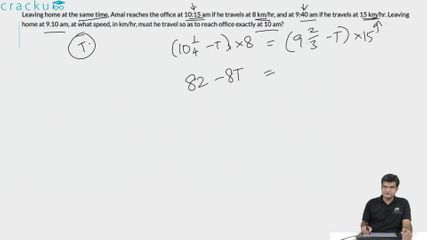Question 18

# Leaving home at the same time, Amal reaches the office at 10:15 am if he travels at 8 km/hr, and at 9:40 am if he travels at 15 km/hr. Leaving home at 9.10 am, at what speed, in km/hr, must he travel so as to reach office exactly at 10 am?

Solution

The difference in the time take to traverse the same distance $$'d'$$ at two different speeds is 35 minutes. Equating this: $$\frac{d}{8}-\frac{d}{15}\ =\ \frac{35}{60}$$

On solving, we obtain $$d = 10 kms$$. Let $$x kmph$$ be the speed at which Amal needs to travel to reach the office in 50 minutes; then

$$\frac{10}{x}=\frac{50}{60}\ or\ x\ =\ 12\ kmph$$.Hence, Option B is the correct answer.

### View Video Solution